Enter the y values below and then plot onto graph paper

#### 1.   For the graph y = x2 + 6x + 8 when x = −8, −4 and 0. There are also the coordinates (−6, 8), (−2, 0) and (2, 24)

 (a) Using a table work out the values of y

y = x2 + 6x + 8

 x −8 −4 0 x2 64 16 0 +6x −48 −24 0 +8 +8 +8 +8 y 24 0 8

 (b) The coordinates of the above are (−8, 24), (−6, 8), (−4, 0), (−2, 0), (0, 8) and (2, 24)

 (c) Plot the above coordinates and draw a line through these points#### 2.   For the graph y = x2 + 9x + 18 when x = −10, −6 and −2. There are also the coordinates (−8, 10), (−4, −2) and (0, 18)

 (a) Using a table work out the values of y

y = x2 + 9x + 18

 x −10 −6 −2 x2 100 36 4 +9x −90 −54 −18 +18 +18 +18 +18 y 28 0 4

 (b) The coordinates of the above are (−10, 28), (−8, 10), (−6, 0), (−4, −2), (−2, 4) and (0, 18)

 (c) Plot the above coordinates and draw a line through these points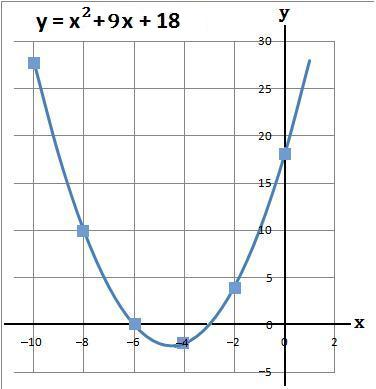#### 3.   For the graph y = x2 + x − 56 when x = −10, 0 and 10. There are also the coordinates (−5, −36) and (5, −26)

 (a) Using a table work out the values of y

y = x2 + x − 56

 x −10 0 10 x2 100 0 100 +x −10 0 +10 −56 − 56 − 56 − 56 y 34 −56 54

 (b) The coordinates of the above are (−10, 34), (−5, −36), (0, −56), (5, −26) and (10, 54)

 (c) Plot the above coordinates and draw a line through these points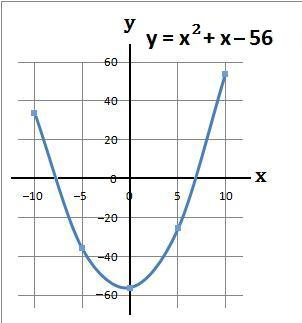#### 4.   For the graph y = x2 + 10x − 24 when x = −15, −5 and 0. There are also the coordinates (−10, −24) and (0, −24)

 (a) Using a table work out the values of y

y = x2 + 10x − 24

 x −15 −5 5 x2 225 25 25 +10x −150 −50 +50 −24 −24 −24 −24 y 51 −49 51

 (b) The coordinates of the above are (−15, 51), (−10, −24), (−5, −49), (0, −24) and (5, 51)

 (c) Plot the above coordinates and draw a line through these points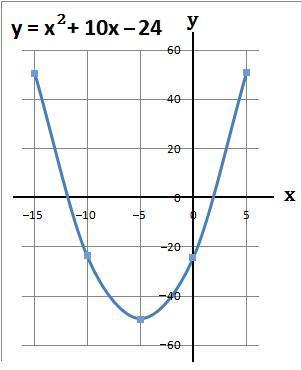#### 5.   For the graph y = x2 − 16x + 60 when x = 2, 8, and 14. There are also the coordinates (4, 12), (6, 0), (10, 0) and (12, 12)

 (a) Using a table work out the values of y

y = x2 − 16x + 60

 x 2 8 14 x2 4 64 196 −16x −32 −128 −224 +60 +60 +60 +60 y 32 −4 32

 (b) The coordinates of the above are (2, 32), (4, 12), (6, 0), (8, −4), (10, 0), (12, 12) and (14, 32)

 (c) Plot the above coordinates and draw a line through these points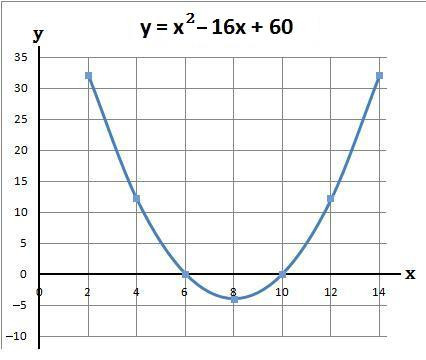#### 6.   For the graph y = x2 − 15x + 36 when x = 0, 6 and 12. There are also the coordinates (2, 10), (4, −8), (8, −20), (10, −14) and (14, 22)

 (a) Using a table work out the values of y

y = x2 − 15x + 36

 x 0 6 12 x2 0 36 144 −15x 0 −90 −180 +36 +36 +36 +36 y 36 −18 0

 (b) The coordinates of the above are (0, 36), (2, 10), (4, −8), (6, −18), (8, −20), (10, −14), (12, 0) and (14, 22)

 (c) Plot the above coordinates and draw a line through these points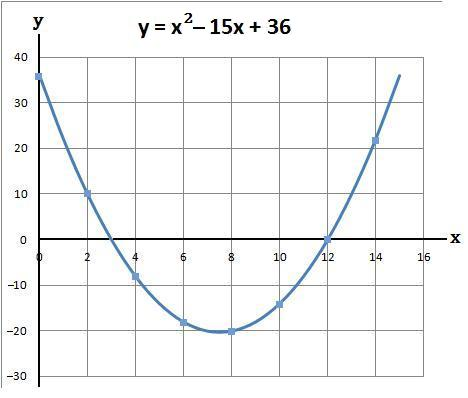#### 7.   For the graph y = x2 − 8x − 48 when x = −5, 5 and 15. There are also the coordinates (0, −48) and (10, −28)

 (a) Using a table work out the values of y

y = x2 − 8x − 48

 x −5 5 15 x2 25 25 225 −8x +40 −40 −120 −48 −48 −48 −48 y 17 −63 57

 (b) The coordinates of the above are (−5, 17), (0, −48), (5, −63), (10, −28) and (15, 57)

 (c) Plot the above coordinates and draw a line through these points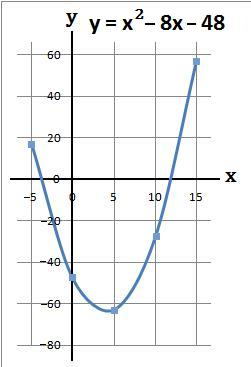back to: